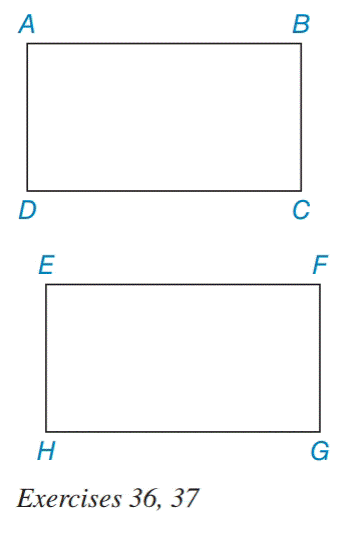Chapter 4.2, Problem 37EElementary Geometry For College St...

7th Edition
Alexander + 2 others
ISBN: 9781337614085

Solutions

Chapter
SectionElementary Geometry For College St...

7th Edition
Alexander + 2 others
ISBN: 9781337614085
Textbook Problem

Are ▱ A B C D and ▱   E F G H congruent if:a) ∠ D and ∠ H are right angles, A B ¯ ≅ E F ¯ , and B C ¯ ≅ F G ¯ ? b) A B ¯ ≅ E F ¯ and ∠ A ≅ ∠ E ?To determine

a)

Whether  ABCD and  EFGH congruent

Explanation

Given,

ABCD and EFGH

AB¯EF¯

BC¯FG

D=H=90° (1)

Since,

D=H=90

The pairs of the opposite angles of a parallelogram are equal.

Thus,

B=90°

F=90°

B=F=90° (2)

Since, a pair of the opposite angles of the parallelograms are at right angle, the other pair of opposite angles are also at right angle

To determine

b)

Whether ABCD and EFGH are congruent

Still sussing out bartleby?

Check out a sample textbook solution.

See a sample solution

The Solution to Your Study Problems

Bartleby provides explanations to thousands of textbook problems written by our experts, many with advanced degrees!

Get Started

Find the domain of each function. 20. (a) g(t)=10r100 (b) g(t) = sin(et 1)

Single Variable Calculus: Early Transcendentals, Volume I

27. Is it true that ln for all positive values of x and y?

Mathematical Applications for the Management, Life, and Social Sciences

Solve the equations in Exercises 126. 10x(x2+1)4(x3+1)510x2(x2+1)5(x3+1)4=0

Finite Mathematics and Applied Calculus (MindTap Course List)

Which curve is simple but not closed?

Study Guide for Stewart's Multivariable Calculus, 8th

True or False: The graph at the right is the graph of a function.

Study Guide for Stewart's Single Variable Calculus: Early Transcendentals, 8th